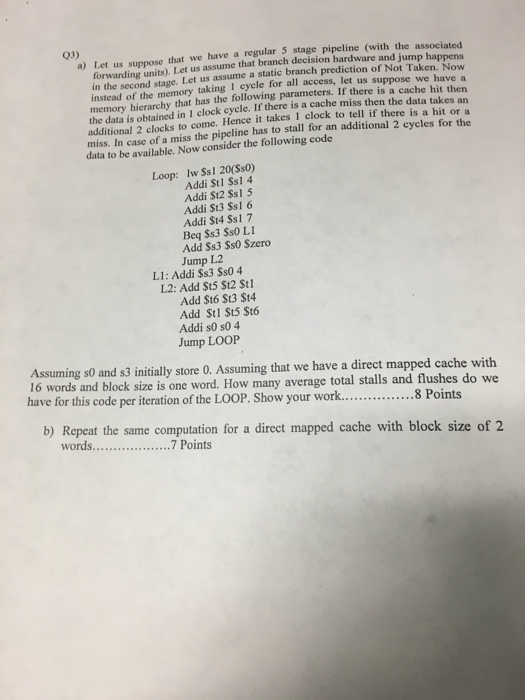# let us suppose that we have a regular 5 stage pipeline with the associated forwardin 5152418Let us suppose that we have a regular 5 stage pipeline (with the associated forwarding units). Let us assume that branch decision hardware and jump happens in the second stage. Let us assume a static branch prediction of Not Taken. Now instead of the memory taking I cycle for all access, let us suppose we have a memory hierarchy that has the following parameters. If there is a cache hit then the data is obtained in 1 clock cycle. If there is a cache miss then the data takes an additional 2 clocks to come. Hence it takes I clock to tell if there is a hit or miss. In case of a miss the pipeline has to stall for an additional 2 cycle for the data to be available. Now consider the following code Loop: lw \$s 1 20(\$s0) Addi \$t 1 \$s1 4 Addi \$t2 \$s1 5 Addi \$t3 \$s1 6 Addi \$t4 \$s1 7 Beq \$s3 \$s0 L1 Add \$s3 \$s0 Szero Jump L2 L1: Addi \$s3 \$s0 4 L2: Add \$t5 \$t2 \$ti Add \$t6 \$t3 \$t4 Add \$t1 \$t5 \$t6 Addi s0 s0 4 Jump LOOP Assuming s0 and s3 initially store 0. Assuming that we have a direct mapped cache with 16 words and block size is one word. How many average total stalls and flushes do we have for this code per iteration of the LOOP. Show your work b) Repeat the same computation for a direct mapped cache with block size of 2 words